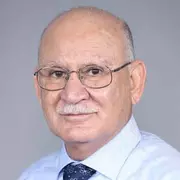X
Local fractional system for economic order quantity using entropy solution
R.W. Ibrahim, H. Jafari, H.A. Jalab,
Published in Springer
2019
Volume: 2019

Issue: 1
Abstract
The economic order quantity (EOQ) model describes the quantity that minimizes the aggregate sum of all cost functions. We propose an assembly for EOQ, nonlinear local fractional differential models with costs functions with respect to time in a cyclic period. For this system, using fractional entropy, we study the related optimization problem and improve a relaxed method for calculating a bounded interval containing the optimal cycle length. Also, for a special class of transportation functions, we study these consequences and introduce processes to compute the optimal size and the matching optimal order stage. © 2019, The Author(s).
••••••